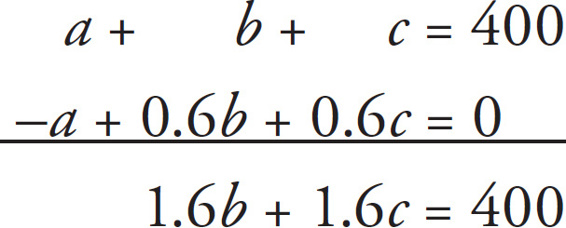# SAT Math Multiple Choice Question 20: Answer and Explanation

### Test Information

Question: 20

6. The sum of three numbers, a, b, and c, is 400. One of the numbers, a, is 40 percent less than the sum of b and c. What is the value of b + c ?

• A. 40
• B. 60
• C. 150
• D. 250